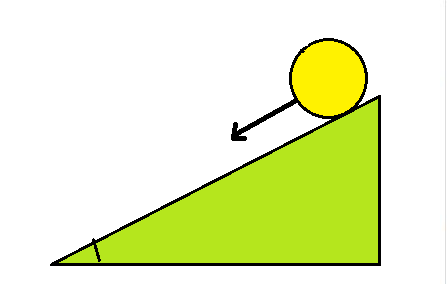# At t=0 a ball, initially at rest, starts to roll down a ramp with constant acceleration. You...

## Question:

At t=0 a ball, initially at rest, starts to roll down a ramp with constant acceleration. You notice it moves 1 foot between t=0 seconds and t = 1 second.1) How far does it move between t = 1 second and t = 2 seconds?

1 foot

2 feet

3 feet

4 feet

6 feet

2) Briefly explain your answer to the previous question

## Uniformly Accelerated Motion:

Any motion can be fully described by the function of displacement vs. time for the object. In the case of constant acceleration, the displacement is related to the time of motion as follows: {eq}x(t) = \dfrac {at^2}{2} {/eq}, where a is the acceleration of motion.

## Answer and Explanation:

The motion is uniformly accelerated one, therefore, for the ball starting from the rest, the equation of motion looks as follows:

{eq}x(t) = \dfrac {at^2}{2} {/eq}

Here

• {eq}a {/eq} is the acceleration of the ball;
• {eq}t {/eq} is the elapsed time;

Coordinate x is along the incline. It is obvious, that the distance covered in 2 seconds is 4 times larger than the distance covered in 1 second. Therefore, the ball moves 4 feet in 2 seconds. If it moves 1 foot in the first second, it moves

{eq}x(2 \ s) - x(1 \ s) = 4 ft - 1 ft = 3 ft {/eq}

Correct answer is 3 ft.

#### Learn more about this topic:Uniformly-Accelerated Motion and the Big Five Kinematics Equations

from AP Physics 1: Exam Prep

Chapter 3 / Lesson 8
66K